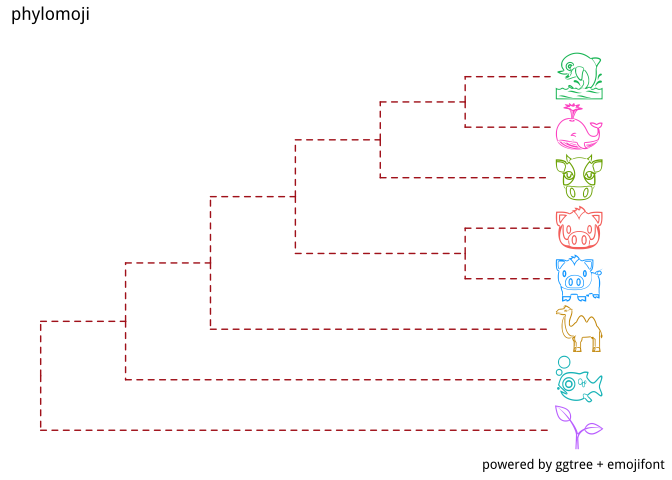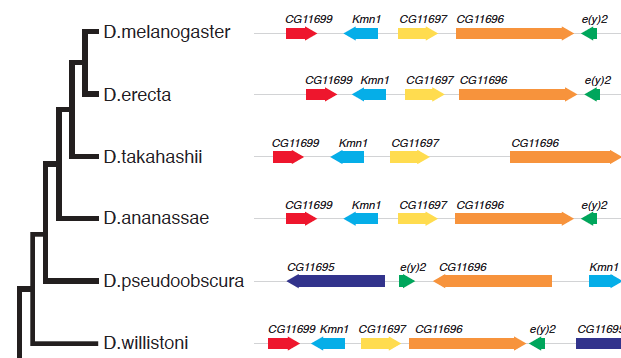# Ggtree

## rename phylogeny tip labels in treeio

I don’t know whether ‘rename taxa’ is a common task or not. It seems not a good idea to rename taxa in Newick tree text, since it may introduce problems when mapping the original sequence alignment to the tree. If you just want to show different or additional information when plotting the tree, it is fine and easy to do it using ggtree: require(treeio) ## Loading required package: treeio require(ggtree) ## Loading required package: ggtree ## ggtree v1.

## Annotating phylogenetic tree with images using ggtree and ggimage

With ggimage, we are able to plot images using grammar of graphics. The layers defined in ggimage can be directly applied to ggtree to annotate phylogenetic tree using local/online image files. ggtree seamlessly work with ggimage. The geom_tiplab and geom_nodelab can accept parameter of geom="image" to parse taxa labels as image files and use them to “label” the taxa using images instead of text strings. Here are some examples for demonstration.

## [Bioc 3.5] NEWS of my BioC packages

I have 8 packages published within the `Bioconductor` project.

A new package treeio was included in BioC 3.5 release.

## Phylomoji with ggtree and emojifont

With ggtree (Yu et al. 2017), it is very easy to create phylomoji. Emoji is internally supported by ggtree.

## Use emoji in taxa labels

``````library(ggtree)
tree_text <- "(((((cow, (whale, dolphin)), (pig2, boar)), camel), fish), seedling);"
x <- read.tree(text=tree_text)
ggtree(x, linetype="dashed", color='firebrick') +
xlim(NA, 7) + ylim(NA, 8.5) +
geom_tiplab(aes(color=label), parse='emoji', size=14, vjust=0.25) +
labs(title="phylomoji", caption="powered by ggtree + emojifont")
``````## convert graphic object to tree object using treeio

I have splitted `ggtree` to 2 packages, `treeio` and `ggtree`. Now `ggtree` is mainly focus on visualization and annotation, while `treeio` focus on parsing and exporting tree files. Here is a welcome message from `treeio` that you can convert `ggtree` output to tree object which can be exported as newick or nexus file if you want.

Thanks to `ggplot2`, output of `ggtree` is actually a `ggplot` object. The `ggtree` object can be rendered as graph by `print` method, but internally it is an object that contains data. `treeio` defines `as.phylo` and `as.treedata` to convert `ggtree` object to `phylo` or `treedata` object.

## add layer to specific panel of facet_plot output

This is a question from ggtree google group:

Dear ggtree team,

how can I apply a geom_xxx to only one facet panel? For example if i want to get `geom_hline(yintersect=1:30)` or a `geom_text()` in the dot panel? I cant see the `facet_grid(. ~ var)` function call, so I don’t know which subsetting to use. I have already read http://stackoverflow.com/questions/29873155/geom-text-and-facets-not-working

``````  tr <- rtree(30)

d1 <- data.frame(id=tr\$tip.label, val=rnorm(30, sd=3))
p <- ggtree(tr)

p2 <- facet_plot(p, panel="dot", data=d1, geom=geom_point, aes(x=val), color='firebrick')
d2 <- data.frame(id=tr\$tip.label, value = abs(rnorm(30, mean=100, sd=50)))

p3 <- facet_plot(p2, panel='bar', data=d2, geom=geom_segment, aes(x=0, xend=value, y=y, yend=y), size=3, color='steelblue') + theme_tree2()
``````

Thanks! Andreas

If this can be done, we can create even more comprehensive tree plots.

## ggtree version of plotTree

PLOTTING TREES + DATA is difficult. @DrKatHolt developed plotTree (R and Python scripts) to visualize associated data with trees, e.g. heatmap, horizontal bar etc.

I reproduced the examples presented in the plotTree repo using ggtree. Source code is freely available in https://github.com/GuangchuangYu/plotTree-ggtree.

Here are the outputs produced by `ggtree`:

## Edge coloring with user data

Coloring edges in ggtree is quite easy, as we can map the color to numerical or categorical values via the `aes(color=VAR)` syntax. For user’s own data, it is also easy as `ggtree` provide the `%<+%` operator to attach user data.

But as it seems not so obviously for `ggtree` users, see question 1, 2, and 3, I will demonstrate how to color edges using user data here.

## align genomic features with phylogenetic tree

A question on biostars asking how to generate the following figure:This can be quite easy to implement in ggtree, I can write a `geom` layer to layout the alignment. As ggbio already provides many `geom` for genomic data and I don’t want to re-invent the wheel, I decided to try `ggtree+ggbio`. This is also the beauty of `R` that packages complete each others.

## xlim_tree: set x axis limits for only Tree panel

A ggtree user recently asked me the following question in google group:

I try to plot long tip labels in ggtree and usually adjust them using xlim(), however when creating a facet_plot xlim affects all plots and minimizes them.

Is it possible to work around this and only affect the tree and it’s tip labels leaving the other plots in facet_plot unaffected?

This is indeed a desire feature, as `ggplot2` can’t automatically adjust `xlim` for text since the units are in two different spaces (data and pixel).